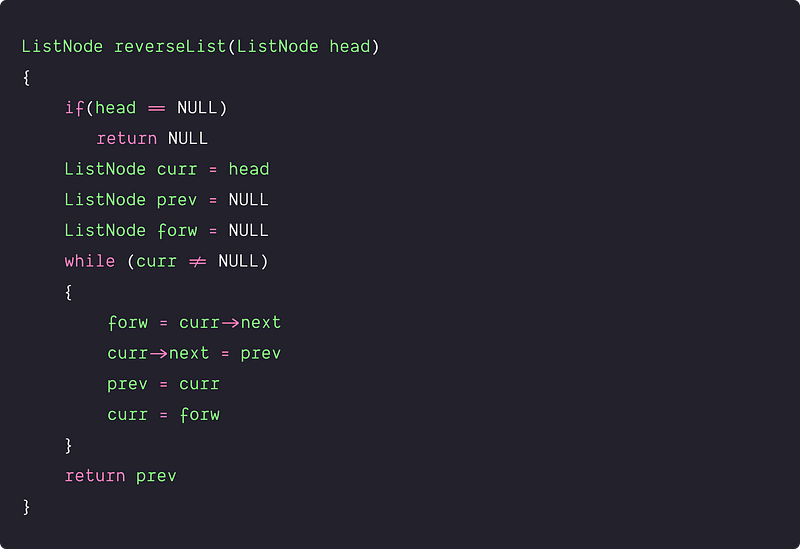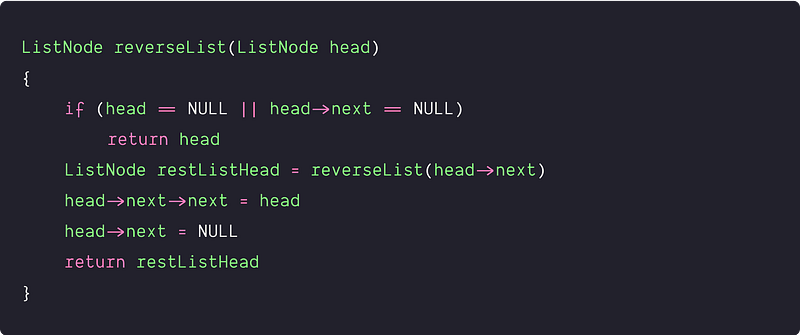Key takeaway

• The problem discussed here is one of the most asked linked list interview questions that can be solved using iteration and recursion.
• This is also a basic problem to improve the understanding of pointer manipulation in a linked list. You can also solve a lot of other interview problems based on this idea.

### Let’s understand the problem

Write a program to reverse a linked list. A head pointer of a linked list is given, and our task is to reverse the entire list so that when the resulted list is traversed it looks like we are traversing the original list from tail to head.

Examples

Input: 1->2->3->4->5->NULL, Output: 5->4->3->2->1->NULL

Input: 1->2->NULL, Output: 2->1->NULL

### Discussed solution approaches

• An iterative approach using three-pointers
• A recursive approach using decrease and conquer

### An iterative approach using three-pointers

Solution Idea

The idea is to reverse the next pointer of each node so that it points to its previous node. During this reversal process, a node does not have reference to its previous node and we must store its previous element beforehand. We also need another pointer to store the next node before changing the reference.

So three-pointers are used :  one for storing the current node, one for the previous node, and the last one for keeping account of the forward node. Always return the new head reference at the end.

Solution Steps

1. Initialize three-pointers prev as NULL, curr as head, and forw as NULL.
2. Iterate through the linked list until the curr becomes NULL and do keep changing the next pointer of each node to its previous node and updating the curr, prev, and forw node :
3. Before changing next of curr, store the forw node i.e forw = curr->next
4. Now change next of curr and point it to prev i.e curr->next = prev
5. Move prev and curr one step forward i.e prev = curr, curr = forw
6. Return the prev node as the new head reference at the end.

Solution PseudocodeTime and space complexity analysis

We are exploring each node once in the while loop and doing constant operation of the pointer movement. so time Complexity = O(n). We are not using any extra space, hence space complexity is O(1).

### A recursive approach using decrease and conquer

Solution Idea

How can we solve this problem using recursion or solution to the problem using the smaller problem?

• The idea is to divide the linked link into two parts : The first node and reversing the rest of the linked list (smaller sub-problem).
• Recursively reverse the rest of the linked list and return the head pointer of this part i.e restListHead. After the reversal, the next node of the head will be the last node of the reversed list.
• For the complete reversal of the list, the head should be the last node. So, do the following operations to ensure this : head->next->next = head, head->next = NULL.
• Return the head pointer of the reversed list i.e. return rest.
• Base Case: if(head == NULL || head->next == NULL) then return Null.

Solution PseudocodeTime and space complexity analysis

We are recursively solving the problem of size n with a smaller sub-problem of size n-1.

• Recurrence relation:T(n) = T(n-1) + c
• The height of the recursion tree is O(n) because the input size is decreasing by 1. At each stage of recursion, we are doing the constant operation. Hence time Complexity = O(n)
• Space Complexity = O(n), for recursion stack space. (Think!)

### Critical ideas to think!

• Can we optimize the 1st approach and solve it using only 2 pointers?
• Can we use a stack for reversing the linked list?
• How the space complexity of the recursive solution is O(n)?

### Comparison of time and space complexities

• Iterative approach: Time = O(n), Space = O(1)
• Recursive approach: Time = O(n), Space = O(n)

### Suggested problems to practice

• Reverse a Linked List from position M to N.
• Reverse a Linked List in groups of a given size.
• Reverse a Linked List using Stack.
• Reverse a circular linked list
• Reverse the first k elements of the given linked list.
• Reverse a doubly linked list

Enjoy learning, Enjoy problem-solving, Enjoy algorithms!

Get well-designed application and interview centirc content on ds-algorithms, machine learning, system design and oops. Content will be delivered weekly.